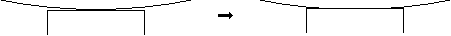Select Page

The program HertzWin developed by Vink System Analysis & Design is used by many people. Sometimes these users have questions about the accuracy and use of the HertzWin results. Some of these questions are discussed here.

## Does the program contain calculation errors (bugs)?

In addition to extensive internal checks, various users have tested HertzWin and compared it with their own calculations. ASML, for example, subjected HertzWin to an extensive test program. All these tests have revealed no errors in the results. Of course, this does not guarantee that HertzWin is 100% error free. It is always wise to verify that the results are what you would expect based on a rough estimate.

## Proper Use of Results

Although the HertzWin program is easy to use, the results must be carefully examined. It is easy to make mistakes with HertzWin results. One of the most common mistakes is to ignore the calculated contact radius of the point contact. For example::

Suppose you need to calculate the contact stresses of a roller with a curved surface, see the figure below.Narrow roller with and without deformation.

This is an elliptical contact that can be easily calculated using HertzWin. Note, however, that the roller must have a finite width and that the contact width cannot exceed the width of the roller. If the calculated contact width is larger, there is no longer an elliptical contact and Hertz’s formulas no longer apply. In this case, the results of HertzWin are inaccurate.

Another problem is that the Hertz equations assume an infinite length of the roller(s) in line contact. In practice, the roller(s) is (are) of finite length and higher stresses may occur at the ends of the roller(s). Also, the effects of misalignment, which causes uneven stress distribution over the contact area, are not considered..

## How accurate are the formulas derived by Heinrich Hertz?

The accuracy of Heinrich Hertz‘s formulas has been the subject of intensive research. For example, article by Anantha Ram B.S. et all of Aalborg University in Denmark describes a study conducted as part of the development of a wear model. The conclusion of this article is that: “the results of the finite element analysis are in good agreement with the theoretical results. The difference between the two is <2.5%“. Since the accuracy of finite element analysis is usually about 1-2%, it is safe to assume that Heinrich Hertz’s formulas give very accurate results. Of course, the following conditions must be met:

• the contact surface of both objects is small compared to the radius of the objects;
• contact surfaces are perfectly smooth (no surface roughness);
• materials distort linearly elastic and are homogeneous;
• there is no friction.

Note: a commonly used limit for the contact surface dimensions is 10% of the contacting radii. If the contact surface dimensions are within 20% of the contacting radii, the results of HertzWin will be less accurate, but still acceptable in many cases.

You can download and use HertzWin free of charge from this website after registration.